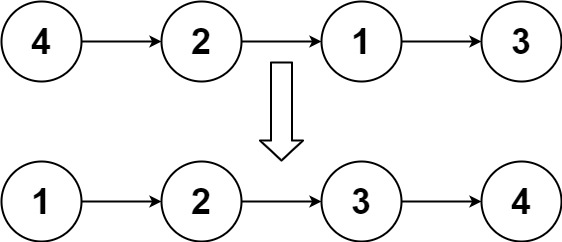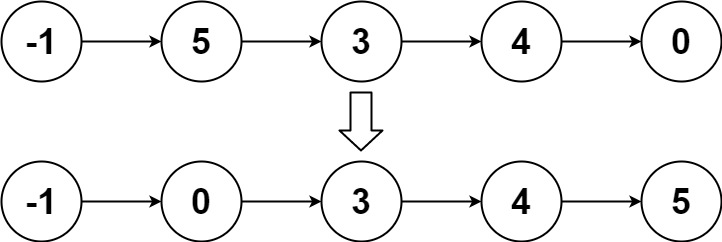# GeetCode Hub

Given the `head` of a linked list, return the list after sorting it in ascending order.

Follow up: Can you sort the linked list in `O(n logn)` time and `O(1)` memory (i.e. constant space)?

Example 1:```Input: head = [4,2,1,3]
Output: [1,2,3,4]
```

Example 2:```Input: head = [-1,5,3,4,0]
Output: [-1,0,3,4,5]
```

Example 3:

```Input: head = []
Output: []
```

Constraints:

• The number of nodes in the list is in the range `[0, 5 * 104]`.
• `-105 <= Node.val <= 105`

/** * Definition for singly-linked list. * public class ListNode { * int val; * ListNode next; * ListNode() {} * ListNode(int val) { this.val = val; } * ListNode(int val, ListNode next) { this.val = val; this.next = next; } * } */ class Solution { public ListNode sortList(ListNode head) { } }